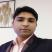# Vector Algebra: Overview, Questions, Preparation

Vector Algebra 2021 ( Vector Algebra )

2.4K ViewsRachit Kumar SaxenaManager-Editorial

Updated on Jun 29, 2021 03:46 IST
1. What is Vector Algebra?
2. Illustrative Example of Vector Algebra
3. FAQs on Vector Algebra

## What is Vector Algebra?

Vector algebra is an important topic of the Class XII Maths subject as it deals with symbols, letters, numbers, and variables. These concepts are later used in equations, formulae, and expressions. In vector algebra, the elements used are denoted as vectors. A vector algebra has some rules and the algebraic operations are performed on the vectors. The vector algebra section carries a weightage of around 14 marks in the examination.

Types of Vectors:

Below mentioned are the different types of vectors:

• Zero Vector
• Unit Vector
• Coinitial Vector
• Equal Vectors
• Negative of A Vector

What is the Definition of Vector?

A vector is an object that has direction and magnitude. An arrow is used for representing the direction and length shows the magnitude. Arrow is on the head of the vector, V, and the magnitude is represented as │V│. Any two vectors whose magnitudes and directions are equal are said to be equal. The arithmetic operations such as addition, multiplication, and subtraction can be performed on vectors.

Addition of Vectors – It has two basic laws. In the following example, there are two vectors P and Q:

Associative Law: P+(Q+R) = (P+Q)+R

Commutative Law: P+Q = Q+P

Multiplication of Vectors – In an equation if k is a scalar quantity and A is the vector, then the multiplication is represented as kA.

Subtraction of Vectors – P–Q = P+(-Q), in this formula the direction of the vectors is reversed but the magnitude remains the same.

A vector uses two terminologies which are a dot product and a cross product.

Dot Product – It is represented by a (.) between two vectors, and is also known as the scalar product. In this method, the two coordinates are of equal length and are multiplied to get a

single number. If P and Q are the two vectors then the dot product can be represented as:

P.Q = │P││Q cosƟ

Cross Product – Across product between two products is denoted by ‘x’. If P and Q are the two vectors, then the cross product is represented as below:

P x Q = │P││Q│sinƟ

## Illustrative Example of Vector Algebra

1. Calculate the dot product of P (1, 3, -4) and Q (3, -5, 2) vectors?

Solution.

As per the dot product formula: P.Q = P1Q1 + P2Q2 + P3Q3 + and so on

Thus, P.Q = 1 . 3 + 3 . (-5) + (-4) . 2

P.Q = 3 – 15 – 8

P.Q = -20

2. Classify the following concepts as scalar or vectors?

Time

Distance

Force

Solution.

Time: It is a scalar, as it has only magnitude and no direction. For instance, 5 seconds.

Distance: It is a scalar, as it has only magnitude and no direction. For instance, 5 meters.

Force: It is a vector, as it has a magnitude and direction. For instance, 5 N.

3. Classify the following in scalars and vectors?

10 kg

40 watt

2 metres North-West

Solution.

10 kg is a scalar quantity as it has only magnitude.

40 watt is a scalar quantity as it has only magnitude.

2 metres North-West is a vector quantity as it has both direction and magnitude.

## FAQs on Vector Algebra

Q: What are the two types of vector multiplication?

A: The two types of vector multiplication are dot product and cross product.

Q: Which are the important concepts of Vector Algebra?

A: The important concepts for the Class XII examinations of vector algebra are types of vectors, the addition of vectors, and the multiplication of vectors.

Q: What are the different types of vectors?

A: There are various types of vectors, but for class XII the types of vectors to study are as follows:
• Zero Vector
• Unit Vector
• Negative Vector
• Collinear Vector
• Co-initial Vector
• Equal Vector

Q: Which are the two types of physical quantities used in vector algebra?

A: The two types of physical quantities used in vector algebra are scalars and vectors. The scalar has only magnitude and the vectors have both magnitude and direction.

Q: Which are the properties of vector addition?

A: The properties of vector addition are commutative property, associative property, and additive identity.